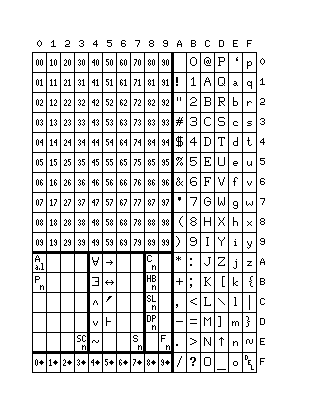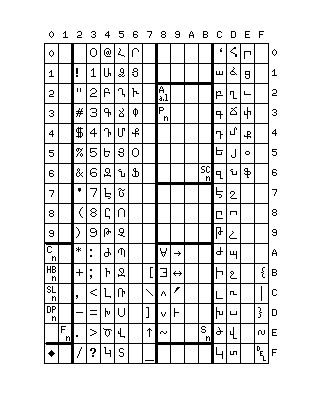[Next] [Up/Previous] [Index]

# A Character Code for Gödel-Numbering

In a later section of these pages, I note that attempting to find a variable-length prefix-property encoding for the natural numbers (the non-negative integers) usually has either the defect that the encoding works only up to a finite limit, or that, in order to reserve space for a symbol indicating the termination of a digit string, the encoding is inefficient by a fixed percentage through its entire length.

However, by combining the simple alternatives having these two different defects, a more complicated coding is possible that has neither; thus, the simple alternatives might be:

• The field may have a fixed length, such as one or two bytes;
• The field may begin with a fixed-length length indicator, such as a one or two byte field giving the number of bytes which contain the number of arguments (this, of course, provides for enormous, if finite, numbers of arguments);
• The field may be expressed in a notation which includes a terminating character, such as being given in ASCII with a terminating null or space, or by being given in BCD with a terminating 1111 nybble.

As noted, these alternatives have the disadvantages noted above; the first two, the finite limit; the last, the constant inefficiency. But a combination of them with a level of overhead that becomes smaller, proportionately, for larger numbers can be constructed as follows:

First, the number of levels may be encoded in decimal digits (or even base-15 digits) terminated with 1111.

If the number of levels is 0, then the number being represented itself is given in the next byte, as a pure binary value.

If the number of levels is 1, then the next byte gives the number of bytes in the number itself, which follows.

If the number of levels is 2, then the next byte gives the number of bytes in the next field, which then gives the number of bytes in the number itself.

And so on and so forth, with only the first field, which indicates the number of levels, not being in pure binary form.

By tolerating the additional inefficiency of starting with decimal digits, rather than digits in base-15, one can achieve something else.

Since the area within ASCII used for printable characters contains 96 characters, or six columns of 16 characters, reserving the ten leading nybbles corresponding to the ten decimal digits still leaves six, enough to allow the presence of all the ASCII printable characters, but with the high bit set.

Thus, a coding like the one below can be achieved:100 symbols are used to represent pairs of decimal digits, having the form nn in hexadecimal.

An additional 10 symbols are used to represent a single decimal digit, followed by an end of string indicator, having the form nF in hexadecimal.

The last six columns of the diagram contain the entire normal printable ASCII character set.

Of course, since no provision is made for representing any control characters, even the carriage return, this is not a useful character coding for general purposes. The 100 code positions used to represent pairs of decimal digits are mostly never used, except to begin the representation of enormously large numbers. This is a scheme of Gödel numbering that happens to make use of ASCII as a handily available source of defined character codings, not a general data communications code which has Gödel numbering added to it. Thus, the coding outlined here is not one amenable to practical purposes.

Fifty character codes remain available. They are shown as divided into three groups in the diagram.

The first group of 20 characters indicates classes of symbols.

The first entry, A(a,l) is used to indicate an abstract letter for use in mathematical formulas; letter l from alphabet a. Thus, A(1,3) might be C, A(2,4) might be d, and so on through italic letters, bold sans-serif letters, outline letters, the Greek alphabet, Fraktur, the Hebrew alphabet, and so on. Both numbers would be indicated in the multiple-level method of indicating numbers, starting with BCD digits, noted above, so that all the alphabets would have an infinite number of letters available, allowing mathematical formulas of arbitrary complexity.

However, this is not the method used to indicate specific alphabetic characters. The code used to indicate a specific character belongs to the third group of charactor codes. Instead, the various alphabets this symbol indicates are to be considered abstract alphabets, each of which posesses an infinite number of letters. Thus, for a particular formula, A(1,1) might stand for x, A(1,2) for y, A(1,3) for z, so that A(1,n) would mean "variable n", and A(2,1) might stand for p, A(2,2) for q, A(2,3) for r, so that A(2,n) would mean "proposition n", and so on. So, if A(n,i) for some n were to indicate the i-th lowercase Greek letter, its real meaning might be "angle n"; although, in that case, A(n,1) would perhaps mean theta, and A(n,2) would mean phi, instead of alpha and beta respectively.

The second entry shown, P(n), indicates variable-length sequences of primes used to suffix a letter in an equation.

The group ends with SC(n), standing for symbol class n; SC(1) stands for A(a,l), and SC(2) stands for P(n), so that if more than 19 entities of the kind represented in this group of 20 characters are required, codes are available. Since n is represented using the code beginning with the BCD representation of the number of levels, it may have any finite value whatever, making an infinite number of codes available.

The second group of 20 characters contains mathematical symbols. The characters of ASCII can also be used, but symbols not found in ASCII are placed here. This section is shown as starting with the symbols for "for all", "there exists", "and", "or", "not", "implies", and "if and only if". While parentheses aren't shown here, because ASCII characters are also available for use in equations, the symbol for "not" is shown, instead of relying on the tilde, because the tilde, belonging to the set of national-use characters associated with the lower-case alphabet, may not always be available.

This group ends with S(n), standing for symbol n; S(1) stands for the symbol for "for all", and again the intent is to make an infinite number of mathematical symbols available.

The third group of 10 characters is used to control selecting alternate character sets to replace the normal ASCII characters. Thus, the Latin alphabet can be replaced by that of Russian or Greek. In the case of Russian, with 32 letters in its alphabet, the tilde would be unavailable, creating the situation noted above.

It is possible to make room for the Armenian alphabet by simply encoding only the first digit, rather than the first two digits, of the decimal number of levels in the representation of an integer in its first byte. In this case, a leading zero would be used when the number of digits is odd, instead of when it is even.

This is only true when numbers are directly inserted into a sequence of characters; where a symbol is used that takes a number as a parameter, the normal notation is used because there is no possibility of using a character instead in the starting position for the number.

The result of this would be a code chart looking like the following:The symbols in the third group, which only spans 10 characters, begin with the following values:

The first entry, C(n), simply means the n-th printable character. Thus, C(32) refers to the space; the conventions used in UNICODE and ISO 10646 and any future applicable standards would be used to identify characters. Again, since the code used to represent n is defined for all natural numbers, future revision to expand the code range will never be required.

The second entry, HB(n), identifies the n-th coding based on high-bit ASCII. Thus, the first diagram, with the printable ASCII characters in the last six columns, would be HB(1). Various national-use variants of 7-bit ASCII would be assigned other values in this code. For Russian and Greek, the principal codes used would have the alphabets of these languages in their normal order, rather than being arranged to have the same codes as Latin letters of similar phonetic value.

The third entry, SL(n), stands for "supplementary lowercase", and refers to arrangements like the one shown above for Armenian.

Once again, the final entry, F(n), allows an infinite number of entities of the current class to exist.

[Next] [Up/Previous] [Index]